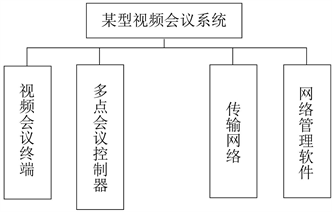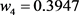﻿ 某型视频会议系统性能的综合评判

# 某型视频会议系统性能的综合评判Comprehensive Evaluation of the Performance of a Video Conference System

Abstract: The main factors affecting the performance of a video conferencing system were analyzed, and an index system for evaluating a video conferencing system was established. The weights of each index were determined by the combination of analytic hierarchy process (AHP) and expert group decision-making method (Delphi). Finally, via grey evaluation theory, a comprehensive evaluation model for the performance of a video conferencing system was validated.

1. 引言Figure 1. Composition of a video conference system

2. 某型视频会议系统评价指标体系的建立

2.1. 指标的确定原则

1) 评估指标应能够反映出某型视频会议系统的本质特性；

2) 指标应尽可能地反映出指标间的相互关联性，同时不能相互包含。

2.2. 某型视频会议系统的评价指标体系Table 1. Performance evaluation index system of a video conference system

3. 确定各层次指标权重

3.1. 确定指标权重的方法

1) 为克服一位专家确定指标权重时带有的主观性问题, 首先采用Delphi法让多位专家同时确定两两指标间的相对重要程度。指标间的相对重要程度按美国运筹学家萨提(Satyr)根据心理学原理提出的1~9标度赋值法  (标度值1、3、5、7、9分别表示甲相对乙同等、稍微、明显、强烈、极端重要)。由此构造

$r\left(r=1,2,\cdots ,s\right)$ 位专家的两两比较判断矩阵： ${C}_{r}={\left({c}_{ij}^{r}\right)}_{n×n}$ ，式中 ${c}_{ij}^{r}$ 表示指标i相对于指标j的重要程度。

2) 对于不同专家的意见，采用几何平均法进行归类处理。参与性能评估的专家共s位，则反应s位专家认为指标i比指标j重要的综合值为： ${a}_{ij}={\left(\underset{r=1}{\overset{s}{\prod }}{c}_{ij}^{r}\right)}^{1/s}$ ，由此得出综合判断矩阵 $a={\left({a}_{ij}\right)}_{4×4}$

4) 求出判断矩阵的最大特征根对应的特征向量，即为评价指标的重要性排序。步骤如下：

① 将矩阵每一列进行归一化处理

$\stackrel{¯}{{a}_{ij}}=\frac{{a}_{ij}}{\underset{k=1}{\overset{n}{\sum }}{a}_{kj}},i,j=1,2,\cdots ,n$

② 将归一化后的矩阵按行相加得： $\stackrel{¯}{{w}_{i}}=\underset{j=1}{\overset{n}{\sum }}\stackrel{¯}{{a}_{ij}},j=1,2,\cdots ,n$

$\stackrel{¯}{{w}_{1}}=1.0707$$\stackrel{¯}{{w}_{2}}=0.8354$$\stackrel{¯}{{w}_{3}}=0.5149$$\stackrel{¯}{{w}_{4}}=1.5789$

③ 将列向量  正规化处理：

${w}_{i}=\frac{\stackrel{¯}{{w}_{i}}}{\underset{j=1}{\overset{n}{\sum }}\stackrel{¯}{{w}_{j}}},i=1,2,\cdots ,n$

${w}_{1}=0.2677$${w}_{2}=0.2089$${w}_{3}=0.1287$，则 $\stackrel{¯}{w}={\left[{w}_{1},{w}_{2},{w}_{3},{w}_{4}\right]}^{T}$ 即为所求的特征向量。

④ 计算矩阵的最大特征值 ${\lambda }_{\mathrm{max}}$

${\lambda }_{\mathrm{max}}=\underset{i=1}{\overset{n}{\sum }}\frac{{\left(AW\right)}_{i}}{n{W}_{i}}$

$AW=\left[\begin{array}{cccc}1& 2& 2.5& 1/3\\ 1/2& 1& 2& 2/3\\ 1/2.5& 1/2& 1& 1/2\\ 3& 1.5& 2& 1\end{array}\right]\left[\begin{array}{l}0.2677\\ 0.2089\\ 0.1287\\ 0.3947\end{array}\right]=\left[\begin{array}{l}1.1388\\ 0.8633\\ 0.5376\\ 1.7685\end{array}\right]$

${\lambda }_{\mathrm{max}}=\underset{i=1}{\overset{4}{\sum }}\frac{{\left(AW\right)}_{i}}{4{W}_{i}}=\frac{1.1388}{4×0.2677}+\frac{0.8633}{4×0.2089}+\frac{0.5376}{4×0.1287}+\frac{1.7685}{4×0.3947}=4.2611$

3.2. 一致性检验

1) 计算判断的一致性检验指标C.I有： $\text{C}.\text{I}=\frac{{\lambda }_{\mathrm{max}}-n}{n-1}$

2) 确定平均一致性指标R.I。可查表3获得。

3) 计算一致性比例C.R。 $\text{C}.\text{R}=\text{C}.\text{I}/\text{R}.\text{I}$ 。当C.R < 0.1时，认为判断矩阵的一致性是可以接受的。反之，当C.R ≥ 0.1时，应该对判断矩阵作适当修正，以保持一定程度的一致性。本判断矩阵中

$\text{C}.\text{I}=\frac{{\lambda }_{\mathrm{max}}-n}{n-1}=\frac{4.2611-4}{4-1}=0.087$

$\text{C}\text{.R}=\text{C}\text{.I}/\text{R}\text{.I}=0.0967<0.1$

3.3. 计算第二层次指标权重Table 4. The weight calculation of the second level index u11, u12 and u13Table 5. The weight calculation of the second level index u21, u22 and u23Table 6. The weight calculation of the second level index u41, u42 and u43

4. 综合评判及实例

4.1. 确定评价等级

4.2. 确定评语集

4.3. 某型视频会议系统性能综合评判

$\begin{array}{c}E={w}_{1}*\left[0.5433\text{\hspace{0.17em}}\text{\hspace{0.17em}}0.21\text{\hspace{0.17em}}\text{\hspace{0.17em}}0.2467\right]*{\left[1\text{\hspace{0.17em}}\text{\hspace{0.17em}}1\text{\hspace{0.17em}}\text{\hspace{0.17em}}1\right]}^{\prime }+{w}_{2}*\left[0.2308\text{\hspace{0.17em}}\text{\hspace{0.17em}}0.5769\text{\hspace{0.17em}}\text{\hspace{0.17em}}0.1923\right]*{\left[1\text{\hspace{0.17em}}\text{\hspace{0.17em}}0.2407\text{\hspace{0.17em}}\text{\hspace{0.17em}}1\right]}^{\prime }\\ +{w}_{3}*\left[0.5\text{\hspace{0.17em}}\text{\hspace{0.17em}}0.5\right]*{\left[1\text{\hspace{0.17em}}\text{\hspace{0.17em}}1\right]}^{\prime }+{w}_{4}*\left[0.5171\text{\hspace{0.17em}}\text{\hspace{0.17em}}0.1243\text{\hspace{0.17em}}\text{\hspace{0.17em}}0.3586\right]*{\left[0.2407\text{\hspace{0.17em}}\text{\hspace{0.17em}}1\text{\hspace{0.17em}}\text{\hspace{0.17em}}0.1728\right]}^{\prime }\\ =0.6364\end{array}$ (式中的转置矩阵部分可根据判断矩阵，再结合白化权函数得到； ${w}_{1}=0.2677$${w}_{3}=0.1287$${w}_{4}=0.3947$ )。

5. 结束语

 电视电话会议系统概括[EB/OL]. https://wenku.baidu.com/view/a2b12dba960590c69ec376a1.html, 2018-07-01.

 一套完整的视频会议系统由哪些构成[EB/OL]. https://wenku.baidu.com/view/361bd30dba1aa8114431d9d5.html, 2018-06-30.

 视频会议终端性能指标[EB/OL]. https://www.docin.com/p-1843995452.html.